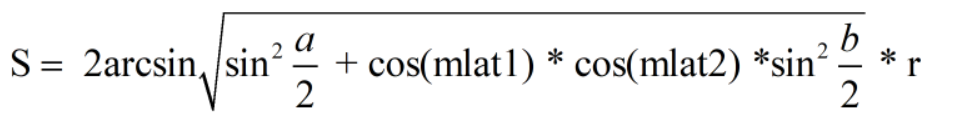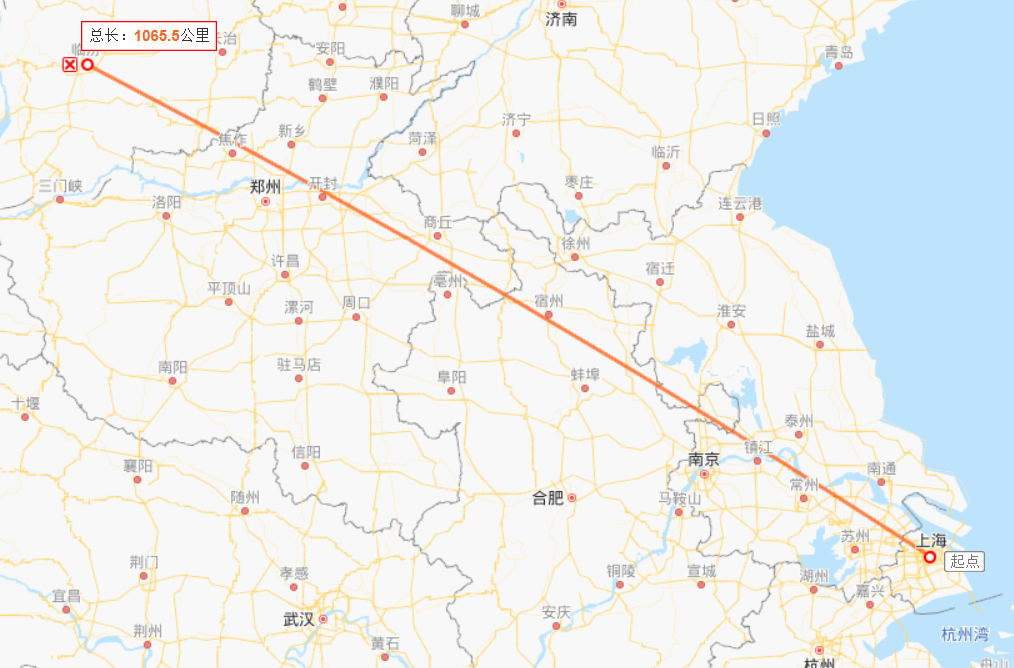• 已知经纬度坐标求两点间距离，用python表示已知地球上任意点（lon1, lat1）,（ lon2, lat2）的经纬度坐标求两点间的距离用haversine表示：1.首先先将经纬度坐标的角度化成弧度（mlon1,mlat1）,(mlon2,mlat2)2....
已知经纬度坐标求两点间距离，用python表示
已知地球上任意两点（lon1, lat1）,（ lon2, lat2）的经纬度坐标，求两点间的距离用haversine表示：
1.首先先将经纬度坐标的角度化成弧度（mlon1,mlat1）,(mlon2,mlat2)
2.利用公式：其中a表示两点维度的差值，即 a = mlat1 - mlat2
其中a表示两点经度的差值，即 b = mlon1 - mlon2
其中r表示地球的半径
用python表示为
def geo_distance(lon1, lat1, lon2, lat2): #地理距离
"""
Calculate the great circle distance between two points
on the earth (specified in decimal degrees)
"""
lon1, lat1, lon2, lat2 = map(radians, map(float, [lon1, lat1, lon2, lat2])) #根据提供的函数对指定序列做映，radians:将角度转换为弧度。
dlon = lon2 - lon1
dlat = lat2 - lat1
a = sin(dlat/2)**2 + cos(lat1) * cos(lat2) * sin(dlon/2)**2
c = 2 * asin(sqrt(a))
r = 6371
return c * r
print(geo_distance(111.5,36.08,121.47,31.23)) #我老家到上海的距离
结果
1067.9651712083848 #单位 km
其实python自带haversine库，可以直接调用，这样就可以不记公式啦！
from haversine import haversine
linfen = (111.5,36.08)
shanghai = (121.47,31.23)
dis = haversine(linfen,shanghai)
print(dis)
结果
1133.5471931316163 #单位 km
可以看出还是有差距的，那那个准确呢？ _用那种方法知道了吧！嘿嘿
展开全文• 类似的需求应该比较多，这里的核心点是关于两个坐标点之间距离的计算，之前没用过这个东西，刚好今天有个这样的需求，记录下。 import requests import psycopg2 import pandas as pd from math import radians, ...
背景：有几个门店和大厦，看看这几个门店和大厦旁边有没有快递柜
数据：门店和大厦只有地址数据，快递柜系统有地址数据和经纬度数据
类似的需求应该比较多，这里的核心点是关于两个坐标点之间距离的计算，之前没用过这个东西，刚好今天有个这样的需求，记录下。
import requests
import psycopg2
import pandas as pd
from math import radians, cos, sin, asin, sqrt

#返回地址的坐标
#使用高德地图的api,获取地址的经纬度，注意key用自己的key,这里的key是瞎写的
base = 'http://restapi.amap.com/v3/geocode/geo'
response = requests.get(base, parameters)
return lon,lat

#返回系统所有的深圳快递柜的坐标
def get_cabinet_coordinate():
conn = psycopg2.connect(database="database",
user="root",
host="localhost",
port="443")
cursor = conn.cursor()
sql = "select  * from  t_station where  city_code = 440300"
cursor.execute(sql)
df = pd.DataFrame(cursor.fetchall())
cursor.close()
df = df.iloc[:,[11,3,4,5,6]]
return df

#获取两个坐标点的距离
def get_distance(lon1, lat1, lon2, lat2):
# 将十进制度数转化为弧度
lon1, lat1, lon2, lat2 = map(radians, [lon1, lat1, lon2, lat2])

# haversine公式
dlon = lon2 - lon1
dlat = lat2 - lat1
a = sin(dlat/2)**2 + cos(lat1) * cos(lat2) * sin(dlon/2)**2
c = 2 * asin(sqrt(a))
r = 6371 # 地球平均半径，单位为公里
#print(c * r * 1000)
return c * r * 1000

if __name__ == '__main__':
df = get_cabinet_coordinate()
lst = list()
#print(lon1,lat1)
for i in df.values:
lon2 = i
lat2 = i
distance = get_distance(lon1, lat1, lon2, lat2)
if distance<=3000:
lst.append({
'lon1':lon1,             #经度
'lat1':lat1,             #维度
'sn':i,               #快递柜sn编码
'lon2':i,             #经度
'lat2':i,             #维度
'distance':distance      #快递柜和分析地址距离
})
#print(dic)
df = pd.DataFrame(lst)
df.to_excel(r'C:\Users\James\Desktop\地域数据_距离.xlsx')
print('数据保存成功')
print(df.head())

展开全文• 已知经纬度坐标求两点间距离，用python表示 已知地球上任意点（lon1, lat1）,（ lon2, lat2）的经纬度坐标求两点间的距离用haversine表示： 1.首先先将经纬度坐标的角度化成弧度（mlon1,mlat1）,(mlon2,mlat2) 2...
已知经纬度坐标求两点间距离，用python表示
已知地球上任意两点（lon1, lat1）,（ lon2, lat2）的经纬度坐标，求两点间的距离用haversine表示： 1.首先先将经纬度坐标的角度化成弧度（mlon1,mlat1）,(mlon2,mlat2) 2.利用公式：其中a表示两点维度的差值，即 a = mlat1 - mlat2 其中a表示两点经度的差值，即 b = mlon1 - mlon2 其中r表示地球的半径

用python表示为
def geo_distance(lon1, lat1, lon2, lat2):  #地理距离
"""
Calculate the great circle distance between two points
on the earth (specified in decimal degrees)
"""
lon1, lat1, lon2, lat2 = map(radians, map(float, [lon1, lat1, lon2, lat2]))  #根据提供的函数对指定序列做映，radians:将角度转换为弧度。
dlon = lon2 - lon1
dlat = lat2 - lat1
a = sin(dlat/2)**2 + cos(lat1) * cos(lat2) * sin(dlon/2)**2
c = 2 * asin(sqrt(a))
r = 6371
return c * r
print(geo_distance(111.5,36.08,121.47,31.23))  #我老家到上海的距离

结果
1067.9651712083848 #单位 km

其实python自带haversine库，可以直接调用，这样就可以不记公式啦！
from haversine import haversine
linfen = (111.5,36.08)
shanghai = (121.47,31.23)
dis = haversine(linfen,shanghai)
print(dis)

结果
1133.5471931316163 #单位 km

可以看出还是有差距的，那那个准确呢？ _用那种方法知道了吧！嘿嘿
展开全文• 根据两个坐标点获取两个坐标点之间的距离，代码奉上，原理不懂… /** * 计算两个坐标点之间的距离，单位米 * @param lat1 //实际坐标点 * @param lng1 //实际坐标点 * @param lat2 //目标坐标点 * @param lng2...
根据两个坐标点获取两个坐标点之间的距离，代码奉上，原理不懂…
	/**
* 计算两个坐标点之间的距离，单位米
* @param lat1	//实际坐标点
* @param lng1	//实际坐标点
* @param lat2 //目标坐标点
* @param lng2  //目标坐标点
* @return 返回的是double类型
*/
public static double getDistance(double lat1, double lng1, double lat2,
double lng2) {
double s = 2 * Math.asin(Math.sqrt(Math.pow(Math.sin(a / 2), 2)
* Math.pow(Math.sin(b / 2), 2)));
s = Math.round(s * 10000d) / 10000d;
s = s * 1000;
return s;
}

return d * Math.PI / 180.0;
}

private  static double EARTH_RADIUS = 6378.137;

展开全文• 本博文源于python基础，旨在探讨python中的lamda表达式应用。内容包含lamda格式/去除列表中偶数/扩大列表中的值/求两坐标距离函数。列表 lambda
• 4，write()方法写入计算的距离 示例代码如下，IDLE环境下可直接运行 import turtle import math x1,y1 = 100,100 x2,y2 = 100,-100 x3,y3 = -100,-100 x4,y4 = -100,100 turtle.penup() turt...海龟绘图 折线图
• 计算两个坐标点之间的距离function calcDistance(lonA,latA,lonB,latB) { var earthR = 6371000; var x = Math.cos(latA * Math.PI / 180.) * Math.cos(latB * Math.PI / 180.) * Math.cos((lonA - lonB) * Math....高德地图
• 一、两个坐标之间距离计算 参考链接： python实现 1、Python 根据地址获取经纬度及求距离 2、python利用地图两个点的经纬度计算两点间距离 　LBS 球面距离公式 美团app筛选“离我最近” 之 地理空间距离计算...
• 话不多说，直接上代码 def haversine(lng1, lat1, lng2, lat2):...# 经度1，纬度1，经度2，纬度2 （十进制度数）计算经纬度坐标点的距离 文章摘自 https://blog.csdn.net/vernice/article/details/46581361
• 今天小编就为大家分享一篇python计算两个地址之间的距离方法，具有很好的参考价值，希望对大家有所帮助。一起跟随小编过来看看吧
• 在直角平面坐标中，我们已知两个坐标点的位置，即物体的上一时刻位置（x1,y1） 和 此时位置（x2,y2） ， 想要知道 该物体的移动方向（直角坐标系的360°角），该如何计算？ 2.解题思路 这是一个可以用反三角函数解的...算法
• 已知不同坐标系的多个坐标点的坐标，求两个坐标系的转换矩阵,是matlab写的程序，三维坐标的。 做机器人视觉导航时用到的这个转换。
• import math ipt = input('请输入x1,y1,x2,y2，以逗号隔开:') data = ipt.split(',') data = [int(x) for x in ...print(data, ',', data, '与', data, ',', data, '之间的距离为：', result)
• 哪位大神知道 已知一个点到两个点的距离和这两个点的坐标，怎么这个点的坐标，用js实现javascript
• # -*- coding: utf-8 -*- # -------------------------------------- # DATE: 2018-12-09 # OUT: calculate_line_distance_amap.py # DEV: yulong # -------------------------------------- import sys ...高德地图
• * 计算两个坐标之间的距离(米) * @param float $fP1Lat 起点(纬度) * @param float$fP1Lon 起点(经度) * @param float $fP2Lat 终点(纬度) * @param float$fP2Lon 终点(经度) * @return int */ function ...
• 求两点间距离 /***** 求两点间距离*****/ float getDistance(CvPoint pointO, CvPoint pointA) { float distance; distance = powf((pointO.x - pointA.x), 2) + powf((pointO.y - pointA.y), 2); distance = ...
• import math def distance(pointx, pointy, x1,y1,x2,y2): a = y2 - y1 b = x1 - x2 x = x2 * y1 - x * y2 dis = (math.fabs(a * pointx + b * pointy + c )) / (math.pow(a * a + b * b, 0.5) ...机器学习 深度学习
• 先给出半正失公式(haversine formula):整理两个式子可得：其中:d为两点的球面距离r为圆的半径，在这里我们假设地球为均匀球体(r=6371.393公里)d/r表示两点在圆上的弧度θφ表示纬度λ表示经度在给出的公式里面，φ、...面积
• 今天小编就为大家分享一篇python实现两个经纬度点之间的距离和方位角的方法，具有很好的参考价值，希望对大家有所帮助。一起跟随小编过来看看吧
• 今天小编就为大家分享一篇Python求两点之间的直线距离(2种实现方法)，具有很好的参考价值，希望对大家有所帮助。一起跟随小编过来看看吧
• 尤其是要会一门编程语言，例如：R语言、Python、Perl等，还要熟悉Linux系统，作为生信小白，听说Python挺简单的，于是就自学了Python，花了天时间了解了Python的基础语法后，今天想做练习题试试手（实践是检验...
• 碰到一道练习题：求解距离矩阵，首先生成一百个二维坐标点，计算任意两个坐标点的距离。我一开始看到这题目是一脸懵逼，然后上网找了一下，更是二脸懵逼。生成一百个二维坐标点，我首先就是想去生成一个1010的二维...numpy
• plt.gca()表示 Get current axis，使用这方法我们可以获得整张图表的坐标对象，这样我们就可以对坐标进行处理了，像移动位置，设置颜色之类的，类似plt.gcf()这是 Get current figure 即获得当前图表的图...Matplotlib
• c++和python实现方式c++python方式一方式二 c++ /*********************...*函数作用： 获取点之间的距离 * *函数参数： *CvPoint2D32f pointO - 起点 *CvPoint2D32f pointA - 终点 * *函数返回值： *double
• Python点集的距离矩阵 使用scipy.spatial的distance，具体代码如下： from scipy.spatial import distance p1=[(1,1),(2,2),(3,3)] p2=[(1,1),(2,2)] p1=np.array(p1,dtype=np.float64) p2=np.array(p2,...scipy
• 使用海龟画图 #导入画图 ...#定义多点的坐标 x1,y1=100,100 x2,y2=100,-100 x3,y3=-100,-100 x4,y4=-100,100 #绘制折线 turtle.penup() turtle.goto(x1,y12) turtle.pwndown() turtle.goto(x2,y2) ...
• 方法一： #导入math包 import math #定义点的函数 class Point: def __init__(self,x=0,y=0): self.x=x self.y=y def getx(self): return self.x def gety(self): return self.y ...
• #定义一个二维平面上的点，包含x轴y轴的坐标以及计算两个点之间距离的方法。 #不一定必须写 import math #定义一个类，用于计算两点之间距离、提供修改坐标函数、提供输出结果函数 class Distance: def __init__...
• 处理地理数据时，经常需要用到两个地理位置间的距离。比如 A 点经纬度（30.553949，114.357399），B点经纬度（129.1344，25.5465）， AB 两点之间的距离。 已知地球上任意两点（lng1，lat1），（lng2， lat2）的...haversine geopy...

# python两个坐标求距离python 订阅